Скачать презентацию Looking at data distributions — Describing distributions with

8f715b7093d4474b0c64cb93547994c4.ppt

• Количество слайдов: 27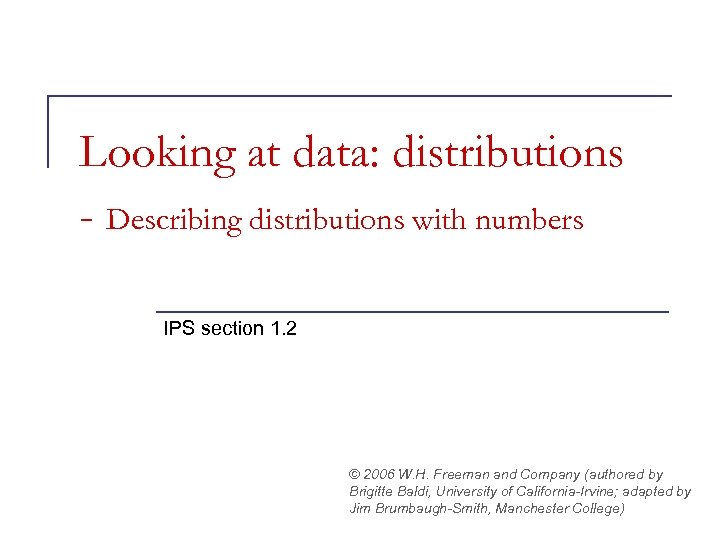Looking at data: distributions - Describing distributions with numbers IPS section 1. 2 © 2006 W. H. Freeman and Company (authored by Brigitte Baldi, University of California-Irvine; adapted by Jim Brumbaugh-Smith, Manchester College)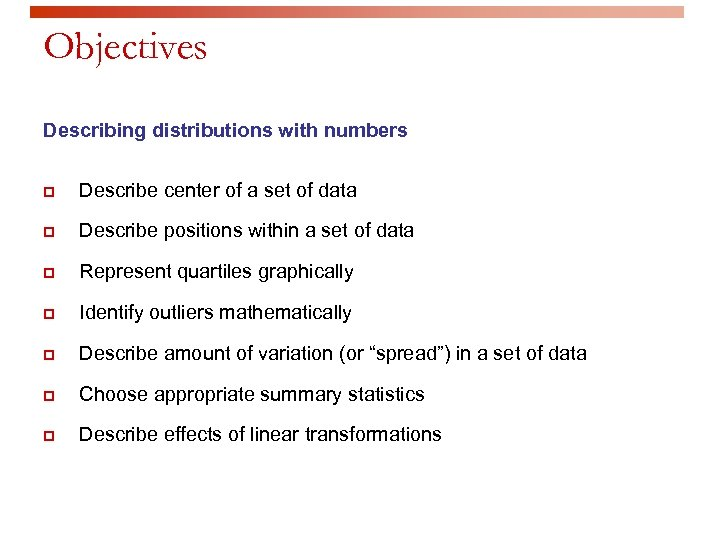Objectives Describing distributions with numbers p Describe center of a set of data p Describe positions within a set of data p Represent quartiles graphically p Identify outliers mathematically p Describe amount of variation (or “spread”) in a set of data p Choose appropriate summary statistics p Describe effects of linear transformations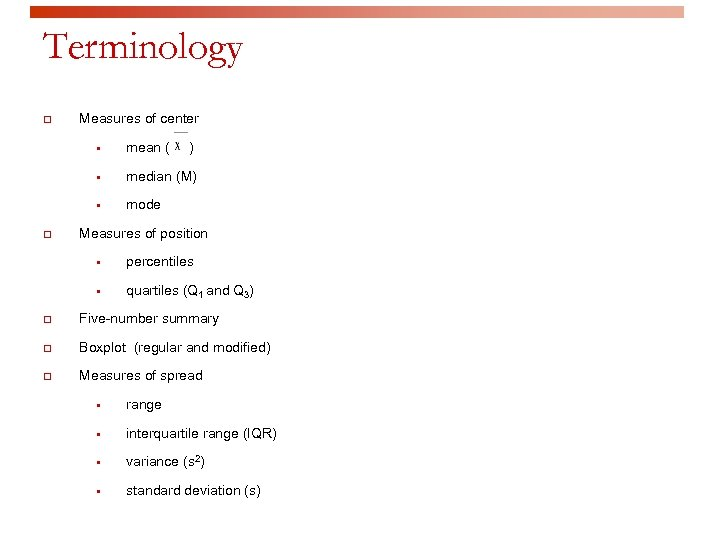Terminology p Measures of center § § median (M) § p mean ( ) mode Measures of position § percentiles § quartiles (Q 1 and Q 3) p Five-number summary p Boxplot (regular and modified) p Measures of spread § range § interquartile range (IQR) § variance (s 2) § standard deviation (s)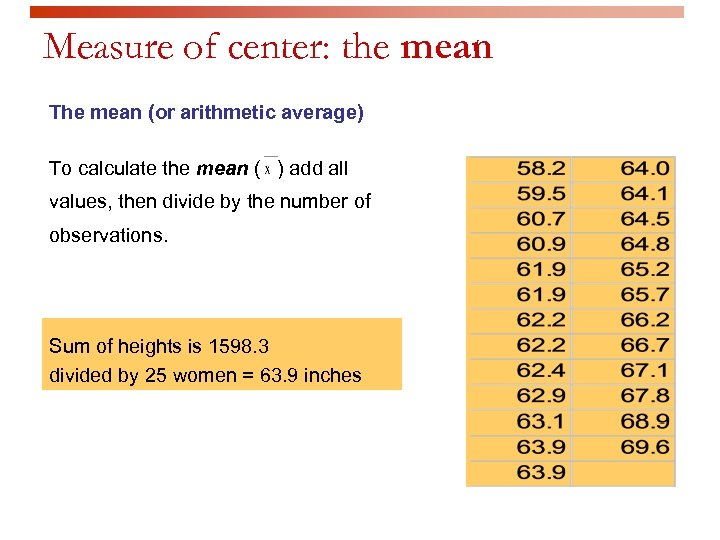Measure of center: the mean The mean (or arithmetic average) To calculate the mean ( ) add all values, then divide by the number of observations. Sum of heights is 1598. 3 divided by 25 women = 63. 9 inches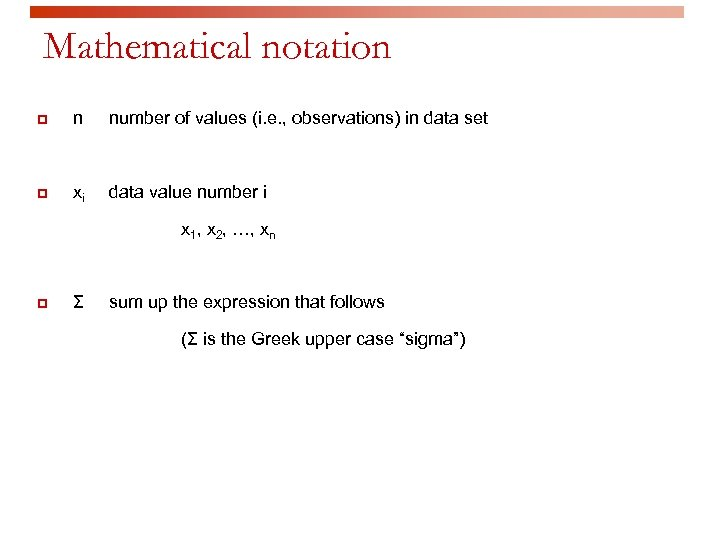Mathematical notation p n number of values (i. e. , observations) in data set p xi data value number i x 1, x 2, , xn p Σ sum up the expression that follows (Σ is the Greek upper case “sigma”)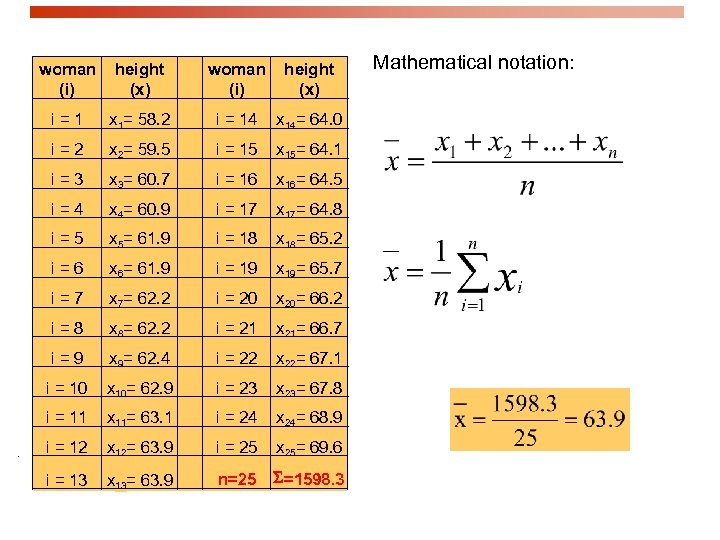woman (i) height (x) i=1 x 1= 58. 2 i = 14 x 14= 64. 0 i=2 x 2= 59. 5 i = 15 x 15= 64. 1 i=3 x 3= 60. 7 i = 16 x 16= 64. 5 i=4 x 4= 60. 9 i = 17 x 17= 64. 8 i=5 x 5= 61. 9 i = 18 x 18= 65. 2 i=6 x 6= 61. 9 i = 19 x 19= 65. 7 i=7 x 7= 62. 2 i = 20 x 20= 66. 2 i=8 x 8= 62. 2 i = 21 x 21= 66. 7 i=9 x 9= 62. 4 i = 22 x 22= 67. 1 i = 10 x 10= 62. 9 i = 23 x 23= 67. 8 i = 11 x 11= 63. 1 i = 24 x 24= 68. 9 i = 12 x 12= 63. 9 i = 25 x 25= 69. 6 i = 13 x 13= 63. 9 n=25 S=1598. 3 Mathematical notation: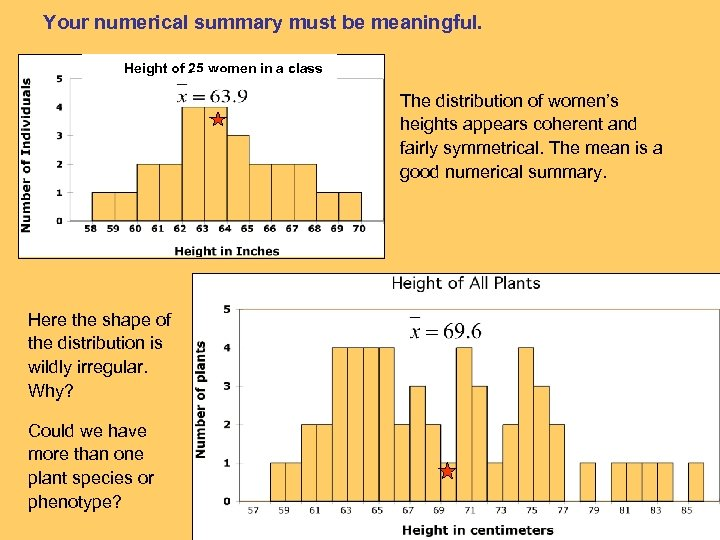Your numerical summary must be meaningful. Height of 25 women in a class The distribution of women’s heights appears coherent and fairly symmetrical. The mean is a good numerical summary. Here the shape of the distribution is wildly irregular. Why? Could we have more than one plant species or phenotype?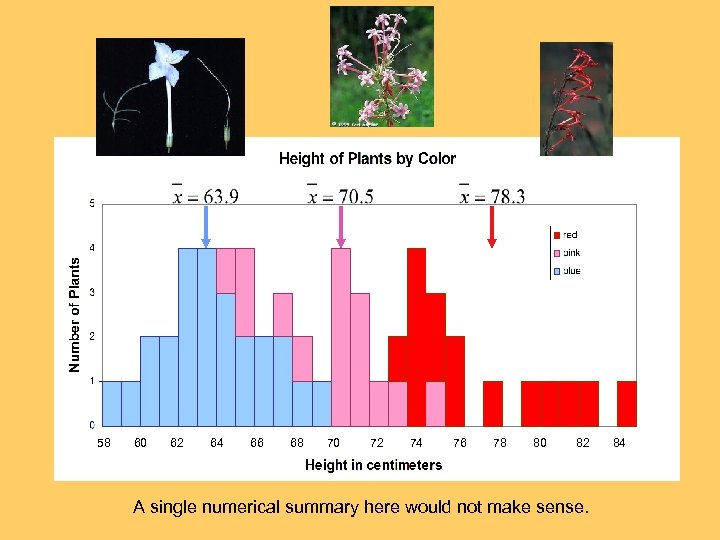58 60 62 64 66 68 70 72 74 76 78 80 82 A single numerical summary here would not make sense. 84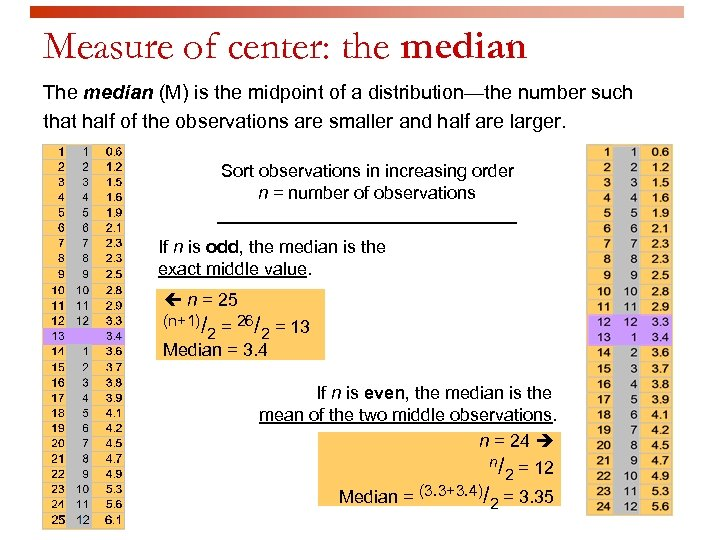Measure of center: the median The median (M) is the midpoint of a distribution—the number such that half of the observations are smaller and half are larger. Sort observations in increasing order n = number of observations _______________ If n is odd, the median is the exact middle value. n = 25 (n+1)/ = 26/ = 13 2 2 Median = 3. 4 If n is even, the median is the mean of the two middle observations. n = 24 n/ = 12 2 Median = (3. 3+3. 4)/2 = 3. 35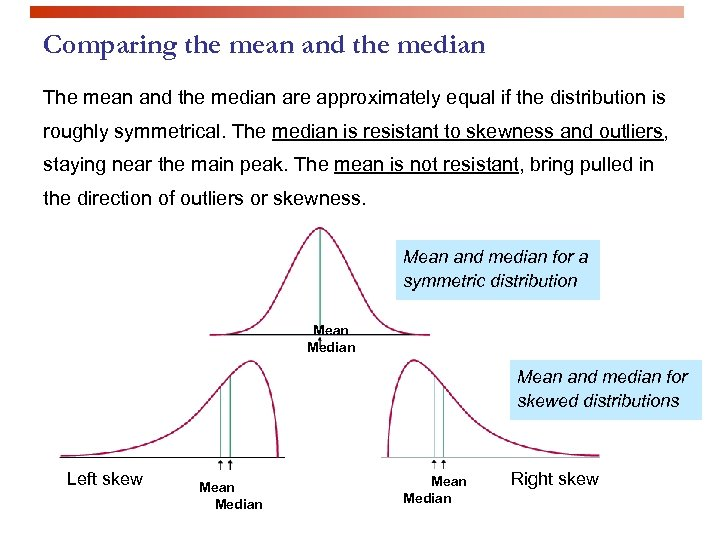Comparing the mean and the median The mean and the median are approximately equal if the distribution is roughly symmetrical. The median is resistant to skewness and outliers, staying near the main peak. The mean is not resistant, bring pulled in the direction of outliers or skewness. Mean and median for a symmetric distribution Mean Median Mean and median for skewed distributions Left skew Mean Median Right skew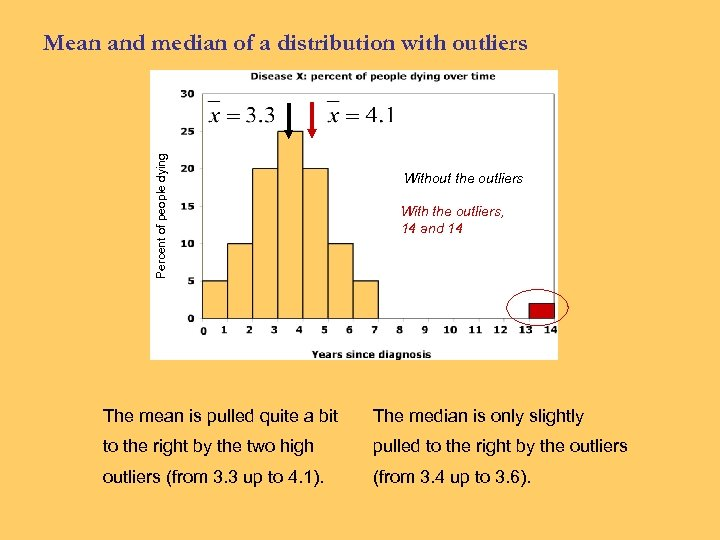Percent of people dying Mean and median of a distribution with outliers Without the outliers With the outliers, 14 and 14 The mean is pulled quite a bit The median is only slightly to the right by the two high pulled to the right by the outliers (from 3. 3 up to 4. 1). (from 3. 4 up to 3. 6).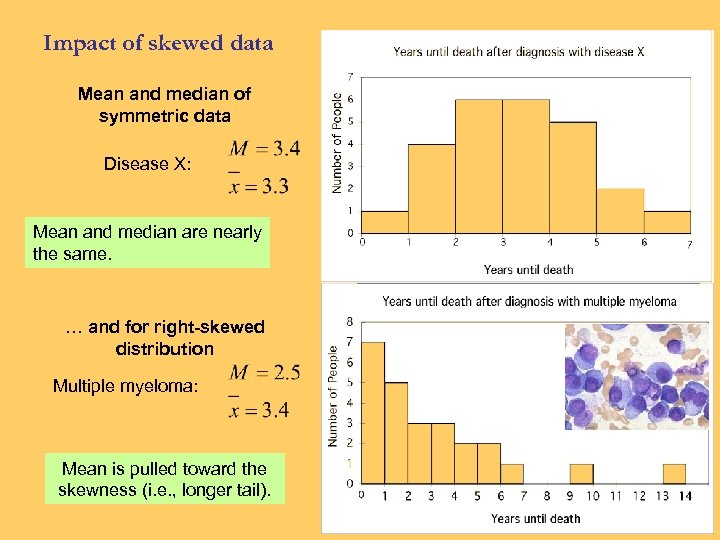Impact of skewed data Mean and median of symmetric data Disease X: Mean and median are nearly the same. … and for right-skewed distribution Multiple myeloma: Mean is pulled toward the skewness (i. e. , longer tail).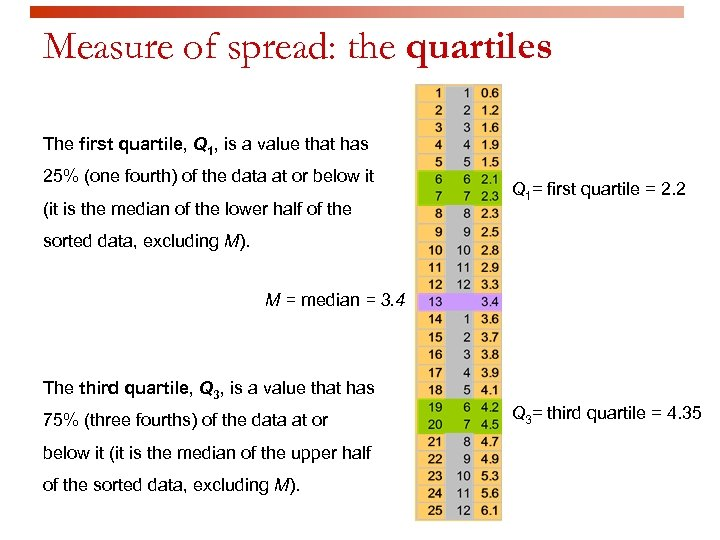Measure of spread: the quartiles The first quartile, Q 1, is a value that has 25% (one fourth) of the data at or below it (it is the median of the lower half of the Q 1= first quartile = 2. 2 sorted data, excluding M). M = median = 3. 4 The third quartile, Q 3, is a value that has 75% (three fourths) of the data at or below it (it is the median of the upper half of the sorted data, excluding M). Q 3= third quartile = 4. 35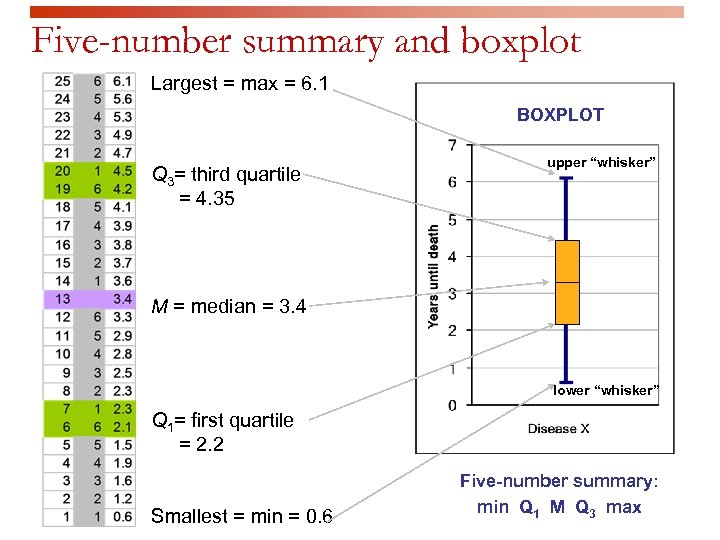Five-number summary and boxplot Largest = max = 6. 1 BOXPLOT Q 3= third quartile = 4. 35 upper “whisker” M = median = 3. 4 lower “whisker” Q 1= first quartile = 2. 2 Smallest = min = 0. 6 Five-number summary: min Q 1 M Q 3 max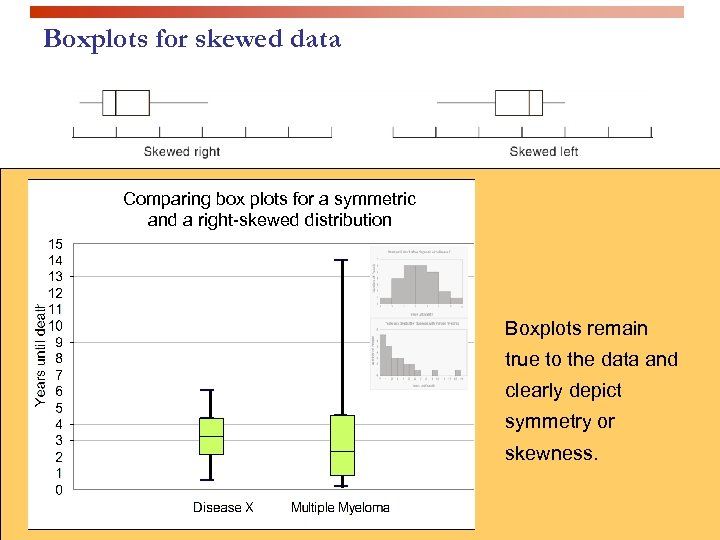Boxplots for skewed data Comparing box plots for a symmetric and a right-skewed distribution Boxplots remain true to the data and clearly depict symmetry or skewness.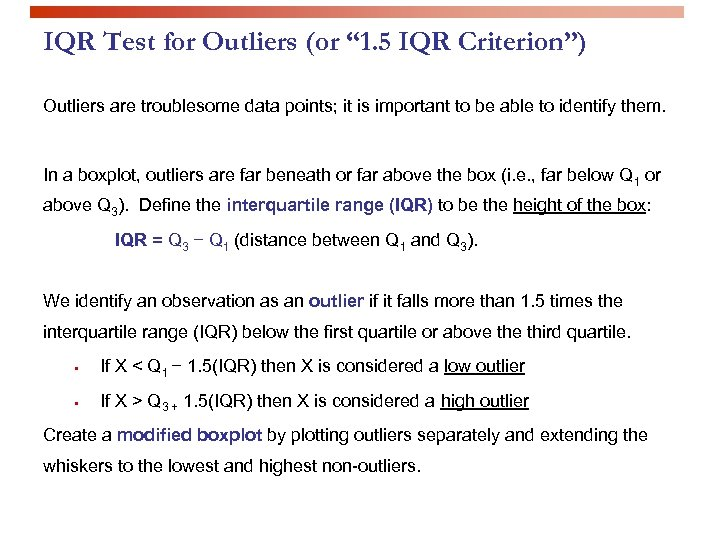IQR Test for Outliers (or “ 1. 5 IQR Criterion”) Outliers are troublesome data points; it is important to be able to identify them. In a boxplot, outliers are far beneath or far above the box (i. e. , far below Q 1 or above Q 3). Define the interquartile range (IQR) to be the height of the box: IQR = Q 3 − Q 1 (distance between Q 1 and Q 3). We identify an observation as an outlier if it falls more than 1. 5 times the interquartile range (IQR) below the first quartile or above third quartile. § If X < Q 1 − 1. 5(IQR) then X is considered a low outlier § If X > Q 3 + 1. 5(IQR) then X is considered a high outlier Create a modified boxplot by plotting outliers separately and extending the whiskers to the lowest and highest non-outliers.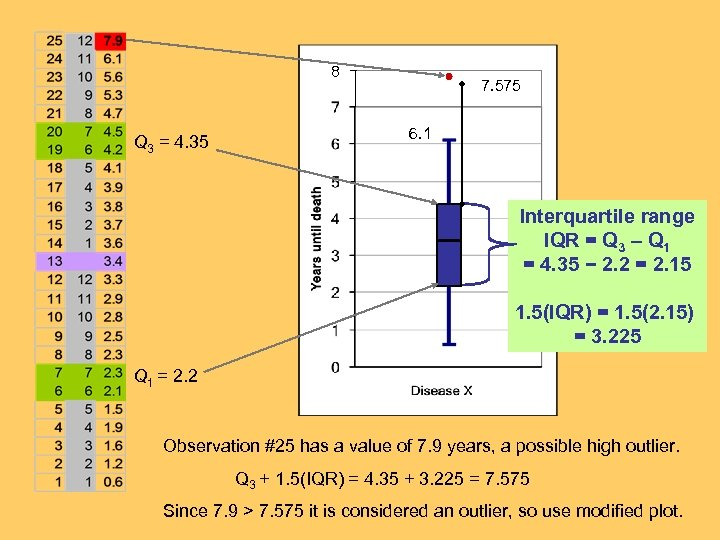8 Q 3 = 4. 35 7. 575 6. 1 Interquartile range IQR = Q 3 – Q 1 = 4. 35 − 2. 2 = 2. 15 1. 5(IQR) = 1. 5(2. 15) = 3. 225 Q 1 = 2. 2 Observation #25 has a value of 7. 9 years, a possible high outlier. Q 3 + 1. 5(IQR) = 4. 35 + 3. 225 = 7. 575 Since 7. 9 > 7. 575 it is considered an outlier, so use modified plot.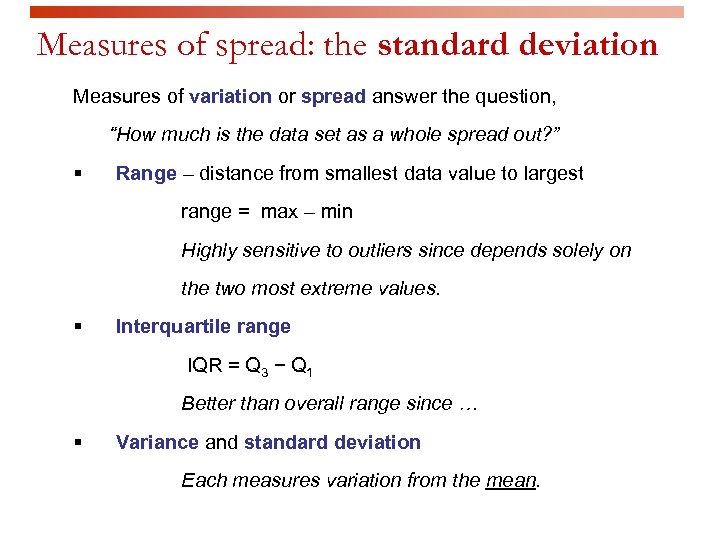Measures of spread: the standard deviation Measures of variation or spread answer the question, “How much is the data set as a whole spread out? ” § Range – distance from smallest data value to largest range = max – min Highly sensitive to outliers since depends solely on the two most extreme values. § Interquartile range IQR = Q 3 − Q 1 Better than overall range since § Variance and standard deviation Each measures variation from the mean.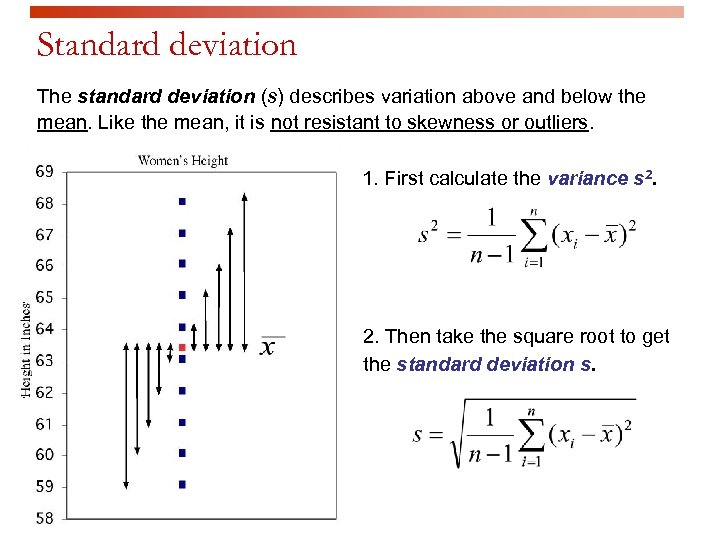Standard deviation The standard deviation (s) describes variation above and below the mean. Like the mean, it is not resistant to skewness or outliers. 1. First calculate the variance s 2. Then take the square root to get the standard deviation s.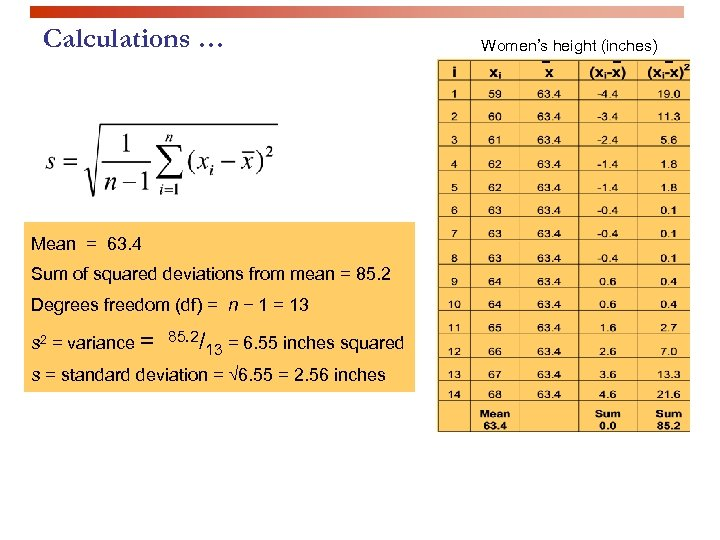Calculations … Mean = 63. 4 Sum of squared deviations from mean = 85. 2 Degrees freedom (df) = n − 1 = 13 s 2 = variance = 85. 2/13 = 6. 55 inches squared s = standard deviation = √ 6. 55 = 2. 56 inches Women’s height (inches)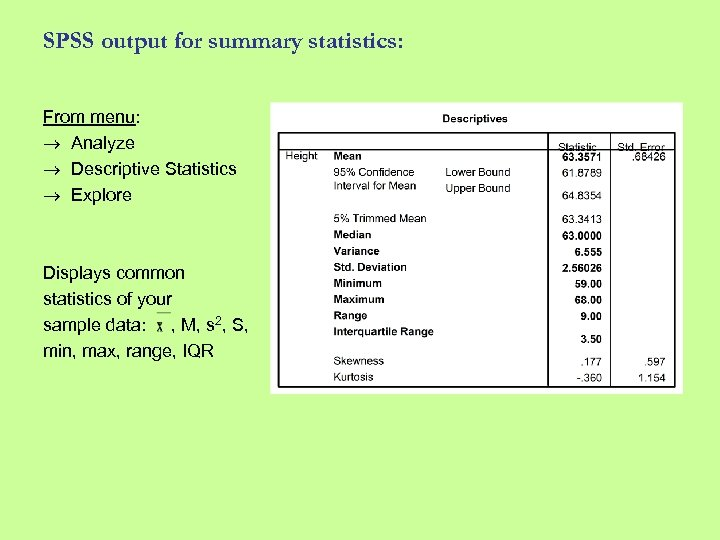SPSS output for summary statistics: From menu: Analyze Descriptive Statistics Explore Displays common statistics of your sample data: , M, s 2, S, min, max, range, IQR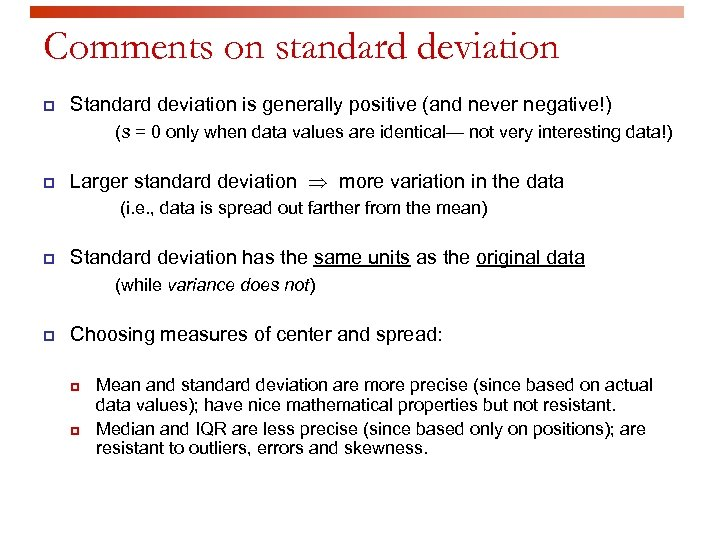Comments on standard deviation p Standard deviation is generally positive (and never negative!) (s = 0 only when data values are identical— not very interesting data!) p Larger standard deviation more variation in the data (i. e. , data is spread out farther from the mean) p Standard deviation has the same units as the original data (while variance does not) p Choosing measures of center and spread: p p Mean and standard deviation are more precise (since based on actual data values); have nice mathematical properties but not resistant. Median and IQR are less precise (since based only on positions); are resistant to outliers, errors and skewness.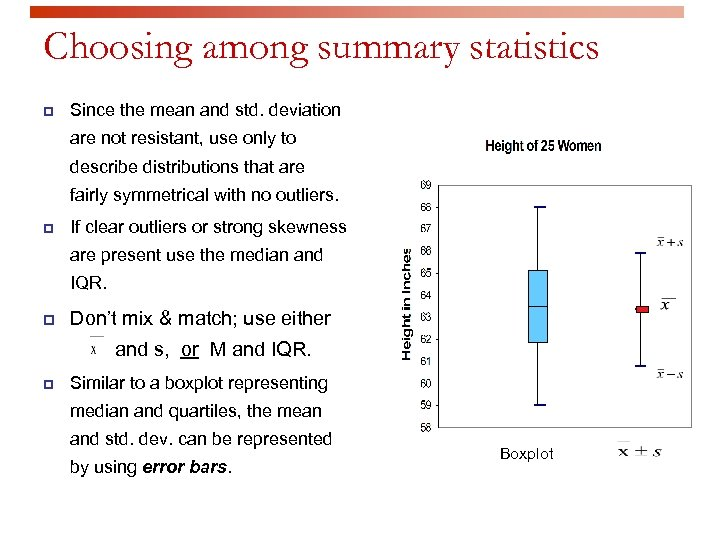Choosing among summary statistics p Since the mean and std. deviation are not resistant, use only to describe distributions that are fairly symmetrical with no outliers. p If clear outliers or strong skewness are present use the median and IQR. p Don’t mix & match; use either and s, or M and IQR. p Similar to a boxplot representing median and quartiles, the mean and std. dev. can be represented by using error bars. Boxplot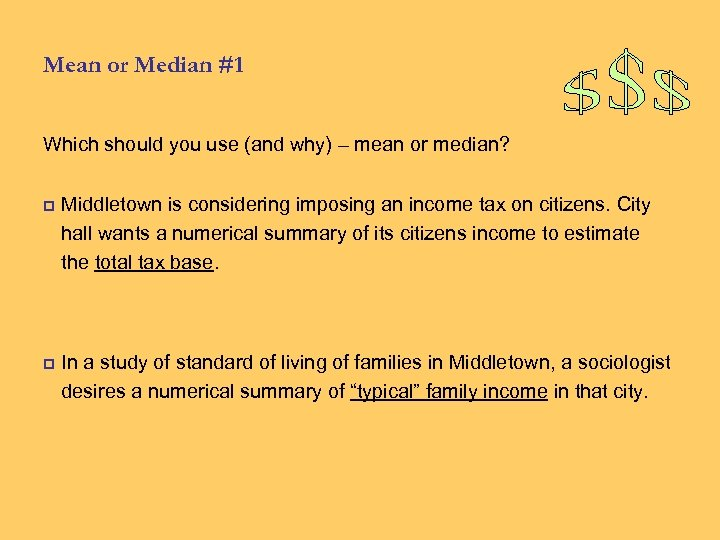Mean or Median #1 Which should you use (and why) – mean or median? p Middletown is considering imposing an income tax on citizens. City hall wants a numerical summary of its citizens income to estimate the total tax base. p In a study of standard of living of families in Middletown, a sociologist desires a numerical summary of “typical” family income in that city.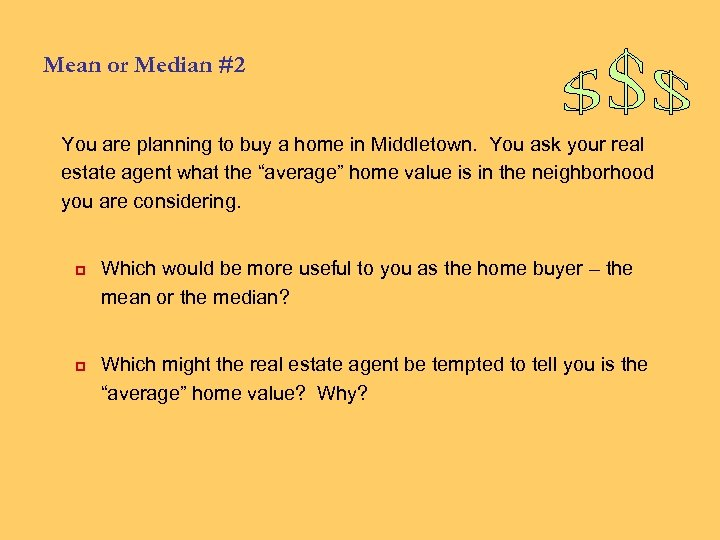Mean or Median #2 You are planning to buy a home in Middletown. You ask your real estate agent what the “average” home value is in the neighborhood you are considering. p p Which would be more useful to you as the home buyer – the mean or the median? Which might the real estate agent be tempted to tell you is the “average” home value? Why?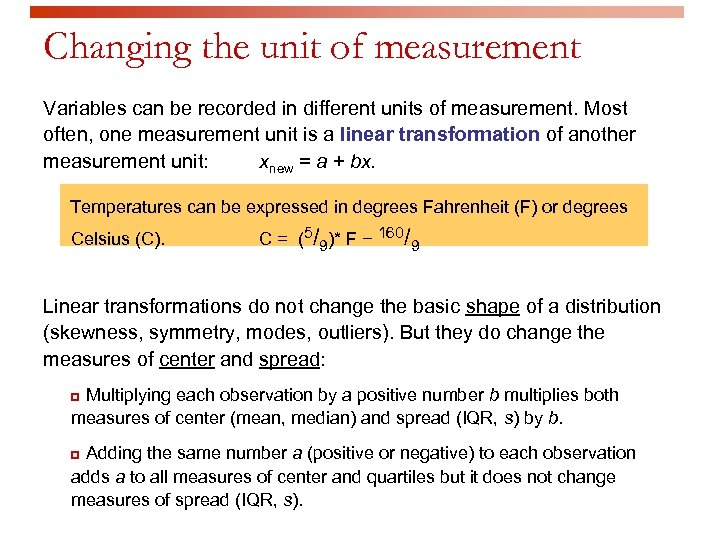Changing the unit of measurement Variables can be recorded in different units of measurement. Most often, one measurement unit is a linear transformation of another measurement unit: xnew = a + bx. Temperatures can be expressed in degrees Fahrenheit (F) or degrees Celsius (C). C = (5/9)* F − 160/9 Linear transformations do not change the basic shape of a distribution (skewness, symmetry, modes, outliers). But they do change the measures of center and spread: Multiplying each observation by a positive number b multiplies both measures of center (mean, median) and spread (IQR, s) by b. p Adding the same number a (positive or negative) to each observation adds a to all measures of center and quartiles but it does not change measures of spread (IQR, s). p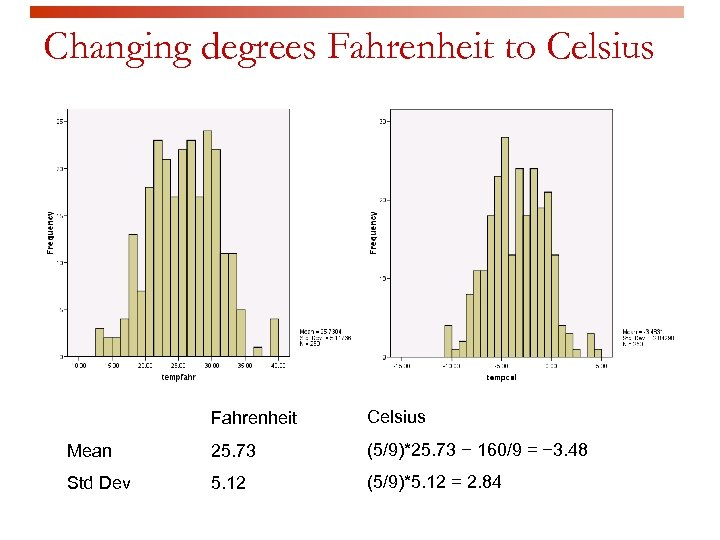Changing degrees Fahrenheit to Celsius Fahrenheit Celsius Mean 25. 73 (5/9)*25. 73 − 160/9 = − 3. 48 Std Dev 5. 12 (5/9)*5. 12 = 2. 84